# Submission

In this lab, there are 6 deliverables. Deliverables 1 to 5 will require pen and paper and are considered individual tasks, while Deliverable 6 is a team task which requires programming.

To submit your solutions create a folder called lab9 and push to your repository with your answers (it can be plain text, markdown, pdf or whatever other format is reasonably easy to read).

### Individual

Please push the deliverables into your personal repository, for math-related questions LaTeX is preferred but handwritten is accepted too.

### Team

Please push the source code for the entire package to the folder lab9 of the team repository. For the plots and discussion questions, please push a PDF to the lab9 folder of your team repository.

Deadline: the VNAV staff will clone your repository on November 10th at midnight.

# 👤 Individual

## 📨 Deliverable 1 - Spy Game [20 pts]

Consider the following spy-style plot of an information matrix (i.e., coefficient matrix in Gauss-Newton’s normal equations) for a landmark-based SLAM problem where dark cells correspond to non-zero blocks: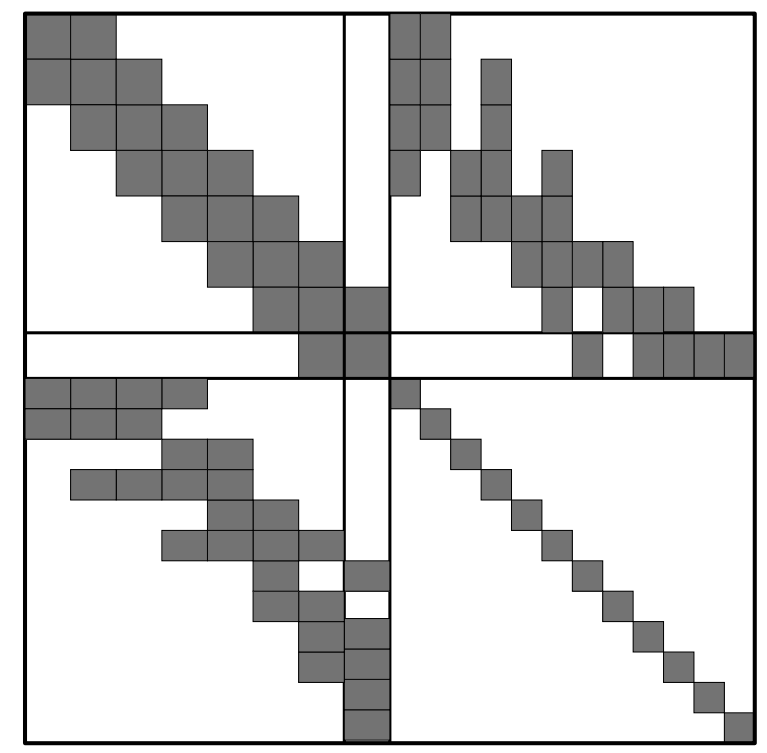Assuming robot poses are stored sequentially, answer the following questions:

1. How many robot poses exist in this problem?
2. How many landmarks exist in the map?
3. How many landmark have been observed by the current (last) pose?
4. Which pose has observed the most number of landmark?
5. What poses have observed the 2nd landmark?
6. Predict the sparsity pattern of the information matrix after marginalizing out the 2nd feature.
7. Predict the sparsity pattern of the information matrix after marginalizing out past poses (i.e., only retaining the last pose).
8. Marginalizing out which variable (chosen among both poses or landmarks) would preserve the sparsity pattern of the information matrix?
9. The following figures illustrate the robot (poses-poses) block of the information matrix obtained after marginalizing out (eliminating) all landmarks in bundle adjustment in two different datasets. What can you say about these datasets (e.g., was robot exploring a large building? Or perhaps it was surveying a small room? etc) given the spy images below?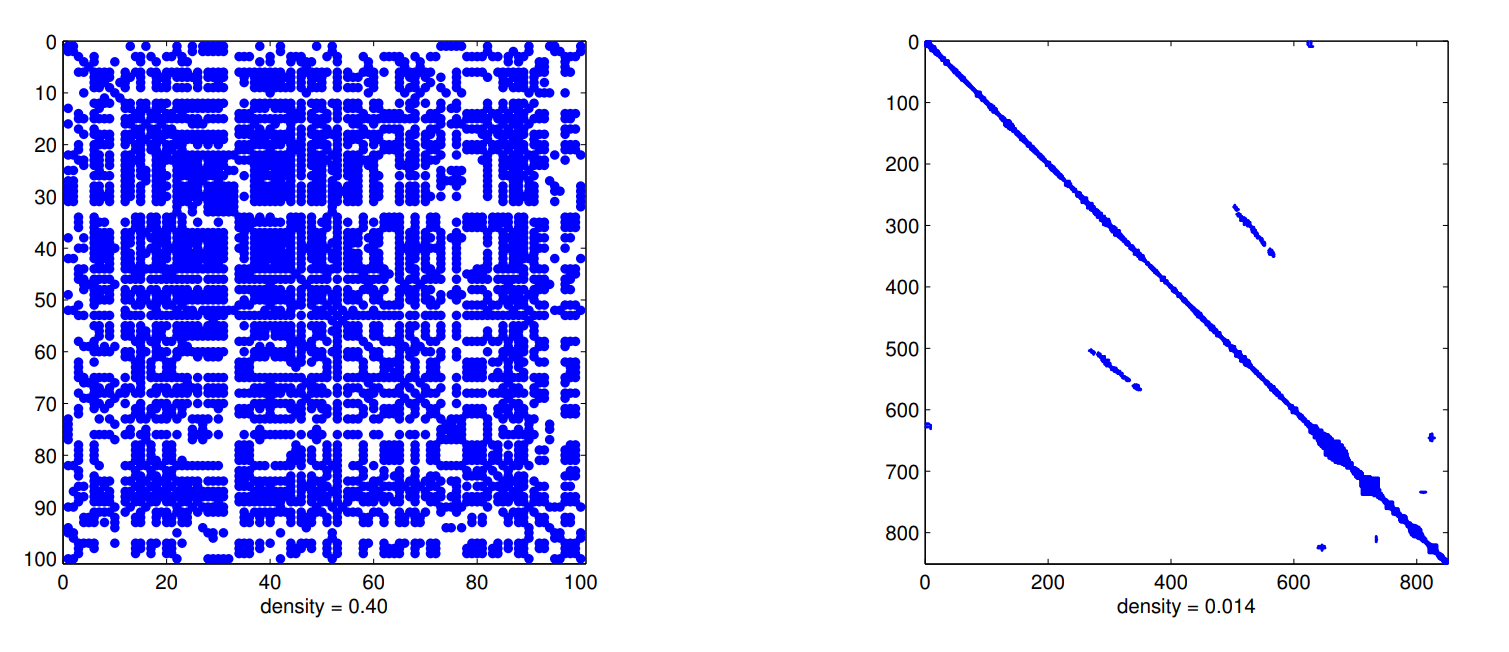## 📨 Deliverable 2 - Well-begun is Half Done [10 pts]

Pose graph optimization is a non-convex problem. Therefore, iterative solvers require a (good) initial guess to converge to the right solution. Typically, one initializes nonlinear solvers (e.g., Gauss-Newton) from the odometric estimate obtained by setting the first pose to the identity and chaining the odometric measurements in the pose graph.

Considering that chaining more relative pose measurements (either odometry or loop closures) accumulates more noise (and provides worse initialization), propose a more accurate initialization method that also sets the first pose to the identity but chains measurements in the pose graph in a more effective way. A 1-sentence description and rationale for the proposed approach suffices.

Hint: consider a graph with an arbitrary topology. Also, think about the problem as a graph, where you fix the “root” node and initialize each node by chaining the edges from the root to that node..

## 📨 Deliverable 3 - Feature-based methods for SLAM [10 pts]

1. Provide a 1 sentence description of each module used by ORB-SLAM (Fig. 1 in the paper can be a good starting point).
2. Consider the case in which the place recognition module provides an incorrect loop closure. How does ORB-SLAM check that each loop closure is correct? What happens if an incorrect loop closure is included in the pose-graph optimization module?

## 📨 Deliverable 4 [Optional] - Direct methods for SLAM [+5 pts]

1. Provide a 1 sentence description of each module used by LSD-SLAM and outline similarities and differences with respect to ORB-SLAM.
2. Which approach (between feature-based or direct) is expected to be more robust to changes in illumination or occlusions? Motivate your answer.

LSD-SLAM is a direct method for SLAM, as opposed to feature-based methods we have worked with previously. As you know, feature-based methods detect and match features (keypoints) in each image and then use 2-view geometry (and possibly bundle adjustment) to estimate the motion of the robot. Direct methods are different in the fact that they do not extract features, but can be easily understood using the material presented in class.

In particular, the main difference is the way the 2-view geometry is solved. In feature-based approaches one uses RANSAC and a minimal solver (e.g., the 5-point method) to infer the motion from feature correspondences. In direct methods, instead, one tries to estimate the relative pose between consecutive frames by minimizing directly the mismatch of the pixel intensities between two images:

$E(\xi) = \sum_i (I\_{ref}(p_i) - I(\omega (p_i, D\_{ref}(p_i), \xi)))^2 = r_i(\xi)^2$

Where the objective looks for a pose $\xi$ (between the last frame $I_{ref}$ and the current frame $I$ that minimizes the mismatch between the intensity $I_{ref}(p_i)$ at pixel $p_i$ for each pixel $p_i$ in the image, and intensity of the corresponding pixel in the current image $I$. How do we retrieve the pixel corresponding to $p_i$ in the current image $I$? In other words, what is this term?:

$I(\omega (p_i, D\_{ref}(p_i), \xi))$

It seems mysterious, but it’s nothing new: this simply represents a perspective projection. More specifically, given a pixel $p_i$, if we know the corresponding depth $D_{ref}(p_i)$ we can get a 3D point that we can then project to the current camera as a function of the relative pose. The $\omega$ is typically called a warp function since it “warps” a pixel in the previous frame $I_{ref}$ into a pixel at the current frame $I$. What is the catch? Well.. the depth $D_{ref}$ is unknown in practice, so you can only optimize $E(\xi)$ if at a previous step you have some triangulation of the points in the image. In direct methods, therefore these is typically an “initialization step”: you use feature-based methods to estimate the poses of the first few frames and triangulate the corresponding points, and then you can use the optimization of $E(\xi)$ to estimate later poses. The objective function $E(\xi)$ is called the photometric error, which quantifies the difference in the pixel appearance in consecutive frames.

NOTE: LDSO (Direct Sparse Odometry with Loop Closures) which you are going to use in Part 2 of this handout is simply an evolution of LSD-SLAM (by the same authors). We are suggesting you to read the LSD-SLAM paper since it provides a simpler introduction to direct methods, while we decided to use LDSO for the experimental part since it comes with a more recent implementation.

## 📨 Deliverable 5 [Optional] - From landmark-based SLAM to rotation estimation [+15 pts]

Consider the following landmark-based SLAM problem:

$\min_{t_i \in \mathbb{R}^3,\ R_i \in \SO{3},\ p_i \in \mathbb{R}^3} \sum_{(i,k) \in \mathcal{E}_l} \| R_i^T(p_k - t_i) - \bar{p}_{ik} \|_2^2 + \sum_{(i,j) \in \mathcal{E}_o} \|R_i^T(t_j - t_i) - \bar{t}_{ij}\|_2^2 + \|R_j - R_i\bar{R}_{ij}\|_F^2$

Where the goal is to compute the poses of the robot $(t_i, R_i),\ i=1,\ldots,N$ and the positions of point-landmarks $p_k, k= 1, \ldots, M$ given odometric measurements $(\bar{t}_{ij}, \bar{R}_{ij})$ for each odometric edge $(i,j) \in \mathcal{E}_o$ (here $\mathcal{E}_o$ denotes the set of odometric edges), and landmark observations $\bar{p}_{ik}$ of landmark $k$ from pose $i$ for each observation edge $(i,k) \in \mathcal{E}_l$ (here $\mathcal{E}_l$ denotes the set of pose-landmark edges).

• Prove the following claim: “The optimization problem (1) can be rewritten as a nonlinear optimization over the rotations $R_i,\ i=1,\ldots,N$ only.” Provide an expression of the resulting rotation-only problem to support the proof.
• Hint: i) Euclidean norm is invariant under rotations, and (ii) translations/positions variables appear …! Consider also using a compact matrix notation to rewrite the sums in the cost function otherwise it will be tough to get an expression of the rotation-only problem.
• The elimination of variables discussed at the previous point largely reduces the size of the optimization problem (from 6N+3L variables to 3N variables).However, the rotation problem is not necessarily faster to solve. Discuss what can make the rotation-only problem more computationally-demanding to solve.
• Hint: What property makes optimization-based SLAM algorithms fast to solve?

# 👥 Team

## Install Docker Engine

Follow the installation instructions listed here. Specifically, you want to follow the instructions listed in the “Install Using the Repository” section.

## Prepare the Dataset

Run the following to download a sequence of the EuRoC dataset into ~/Datasets:

cd ~
wget http://robotics.ethz.ch/~asl-datasets/ijrr_euroc_mav_dataset/machine_hall/MH_01_easy/MH_01      _easy.zip -O Datasets/EuRoC/MH01.zip && unzip Datasets/EuRoC/MH01.zip -d Datasets/EuRoC/MH01 && r      m Datasets/EuRoC/MH01.zip


## ORB-SLAM2

### If you have a working ORB-SLAM2 ROS package …

If you have ORB-SLAM2 installed from Lab 8, you should be able to make it run on the EuRoC dataset that we downloaded previously. You might be already familiar with the visualization window of ORB-SLAM: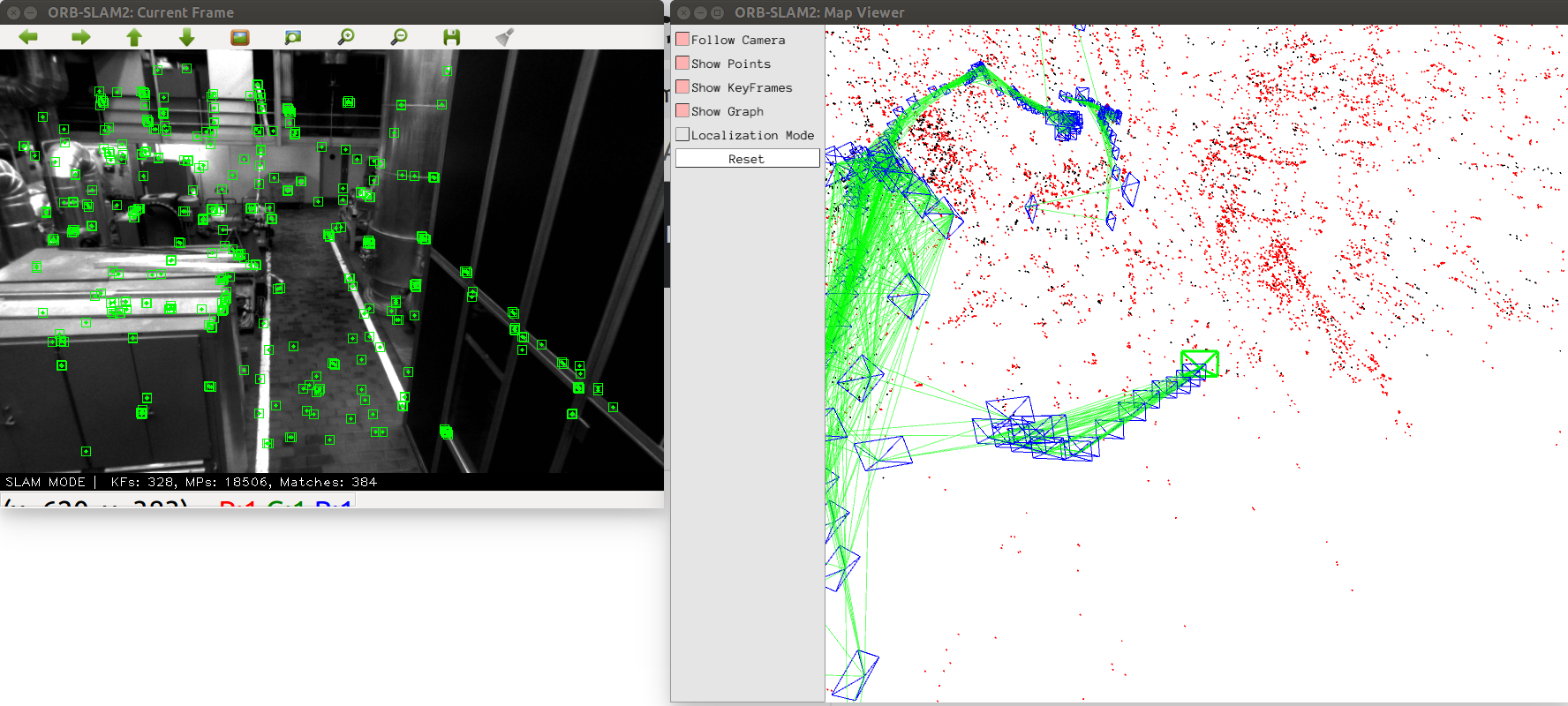Let us now use ORB-SLAM to estimate the trajectory of the camera. For that, run ORB-SLAM for the whole MH_01_easy EuRoC sequence (available here) and wait for the KeyFrameTrajectory_TUM_Format.txt file, which contains the estimated trajectory, to be generated (it will be generated when you ctrl+c the process, which you should only do at the end of the rosbag).

### Otherwise …

You should still be able to get ORB-SLAM2 running by using a Docker container. Run the following command to pull the ORB-SLAM2 container to your local machine:

docker run -it --rm -e DISPLAY=DISPLAY -v /tmp/.X11-unix:/tmp/.X11-unix -v ~/Datasets:/Datasets youyu/orb_slam2:ubuntu18  You will see your terminal command line prompt changes to something like this: root@pc-name:/#  This essentially means that you are currently running the terminal inside the Docker container you just built. With the Docker container running, run the following command: cd /ORB_SLAM2 ./Examples/Stereo/stereo_euroc Vocabulary/ORBvoc.txt Examples/Stereo/EuRoC.yaml /Datasets/EuRoC/MH01/mav0/cam0/data /Datasets/EuRoC/MH01/mav0/cam1/data Examples/Stereo/EuRoC_TimeStamps/MH01.txt  You should see a visualization GUI popping up: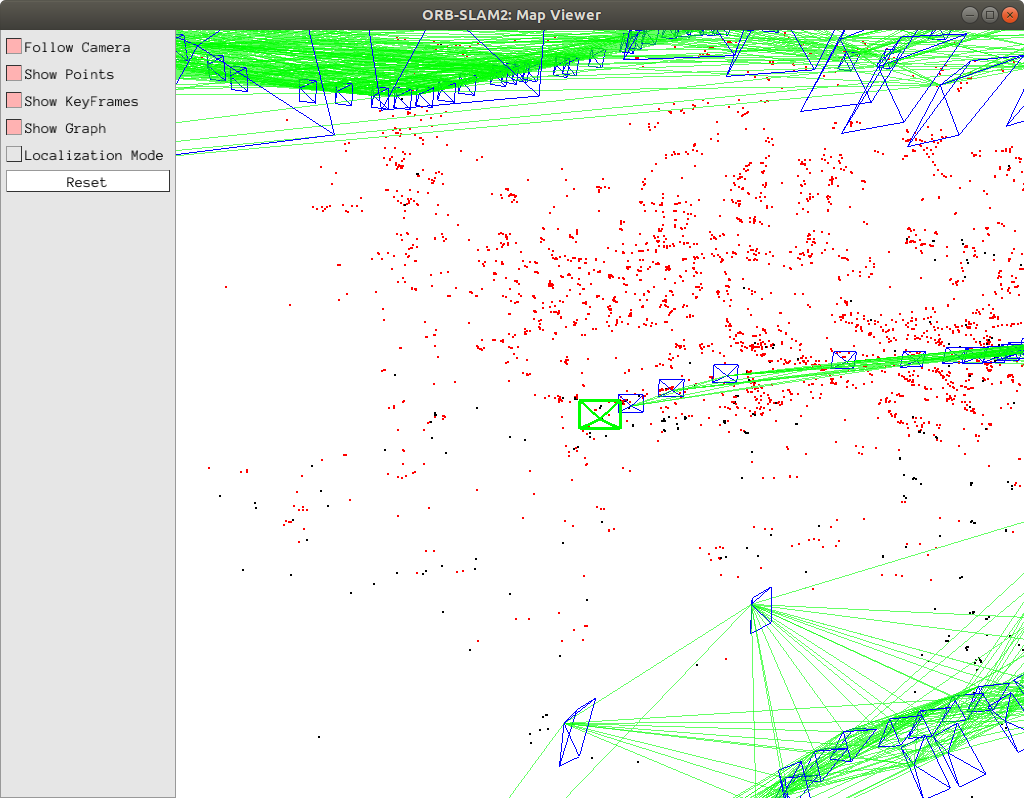When the program finishes, you should see a file named CameraTrajectory.txt. Run the following command to move the file out of the container into your ~/Datasets folder: mv CameraTrajectory.txt /Datasets/orb-slam2-traj.txt  ## ORB-SLAM3 We are also going to try ORB-SLAM3 on the same dataset, so we can compare their performances. ORB-SLAM3, as the name suggests, is the latest version of ORB-SLAM. To evaluate the performance of ORB-SLAM3, instead of installing it as a ROS package, we are going to take a different route: we are going to deploy and test it in a Docker container. ### Get the ORB-SLAM3 Docker Container We will be using an existing Dockerfile for ORB-SLAM3. Run the following instructions: cd ~ # an arbitrary folder outside your ROS workspace git clone https://github.com/jahaniam/orbslam3_docker.git cd orbslam3_docker ./download_dataset_sample.sh build_container_cpu.sh  After the container finishes building, run the following command: docker exec -it orbslam3 bash  You will see your terminal command line prompt changes to something like this: root@pc-name:/ORB_SLAM3#  This essentially means that you are currently running the terminal inside the Docker container you just built. Now, inside the Docker container bash, run the following: cd /ORB_SLAM3/Examples ./Stereo/stereo_euroc ../Vocabulary/ORBvoc.txt ./Stereo/EuRoC.yaml /Datasets/EuRoC/MH01 ./Stereo/EuRoC_TimeStamps/MH01.txt dataset-MH01_stereo  And you should see something like this: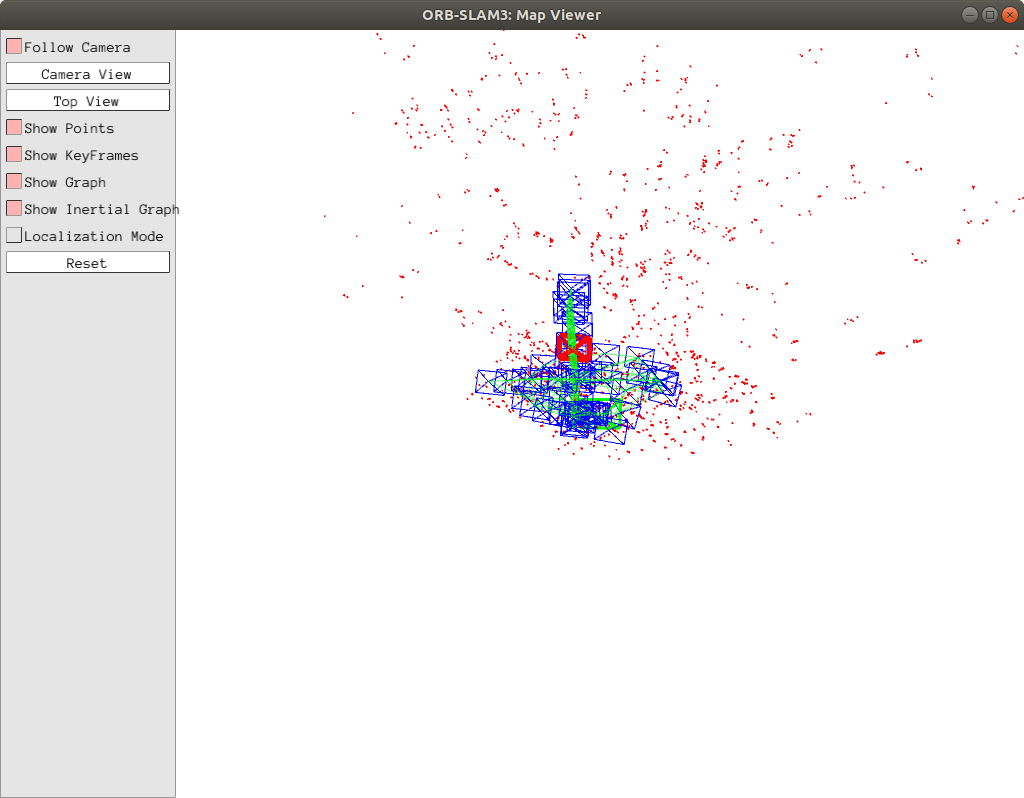Wait until the program finishes the run. You should now see a file named f_dataset-MH01_stereo.txt inside the Examples folder on your local machine. ## 📨 Deliverable 6 - Performance Comparison [60 pts] Download and install the EVO repo, which allows us to evaluate the quality of trajectory estimates. Note that installation may take a while. Feel free to read the useful Wiki page, Readme, and even Jupyter notebooks! that the repo provides. First, we need to fix the timestamps on the ORB-SLAM3 trajectory file. Run the fix_timstamps.py script after replacing the paths inside the script to the paths of your f_dataset-MH01_stereo.txt file. Now you can use the evo_traj tool to plot both trajectories for MH_01_easy. Report this plot and comment on the differences that you see between trajectories: evo_traj tum orb-slam2-traj.txt f_dataset-MH01_stereo_fixed.txt --plot  (Specify the correct path for each of your trajectory files) In general, the trajectories output from different SLAM systems may use different conventions for the world frame, so we have to align the trajectories in order to compare them. This amounts to solving for the optimal (in the sense of having the best fit to the ground truth)\SE{3}transformation of the estimated trajectory. We’ve discussed these types of problems at length in class, and we don’t ask you to implement the alignment process in this lab. Fortunately, tools like evo have already provided efficient implementations that do this for us. Let’s compare to the ground-truth pose data from the EuRoC dataset next, taking into consideration this pose alignment. Grab the ground-truth pose data data.csv from the MH_01_easy.zip file on the EuRoC website (here). The data.csv file is in the EuRoC format, so first use evo_traj to convert to TUM format. evo_traj euroc data.csv --save_as_tum  Next, run: evo_traj tum orb-slam2-traj.txt f_dataset-MH01_stereo_fixed.txt --ref data.tum --plot --align  Report this plot and comment on the differences that you see between trajectories. ## 📨 [Optional] Deliverable 7 - LDSO [+10 pts] Based on LSD-SLAM, LDSO is a direct, yet sparse, formulation for SLAM. It is also capable of handling loop closures. Let us install the code for LDSO, to do that follow the Readme.md in the GitHub repository. In the same Readme.md there is an example on how to run this pipeline for the EuRoC dataset. Detailed below for convenience: cd ~/code/LDSO ./bin/run_dso_euroc preset=0 files=/my_path_to_data/EuRoC/MH_01_easy/mav0/cam0  (Replace my_path_to_data with your path to the data). At this point, you should be able to see the following window: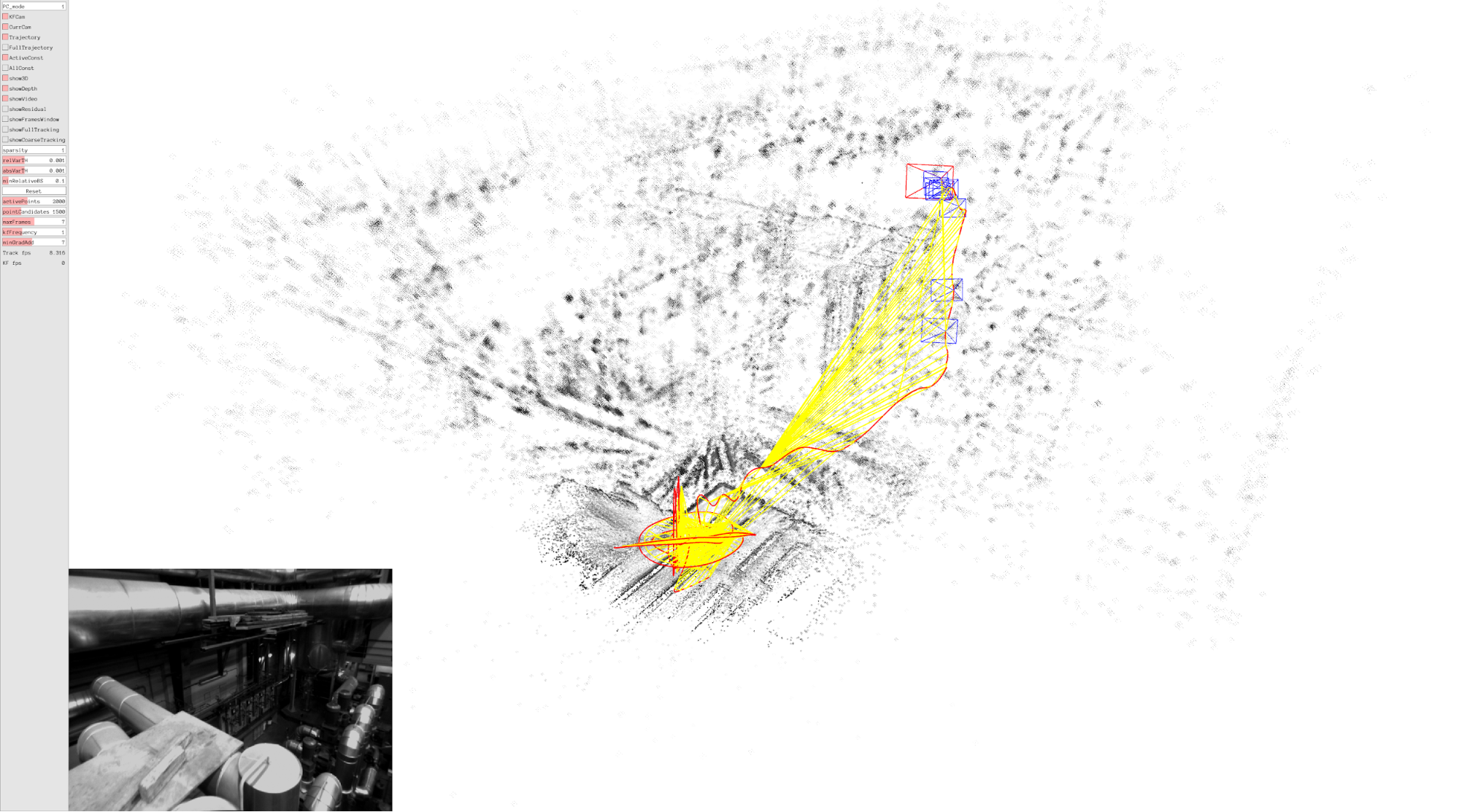LDSO will output by default the results in “./results.txt” file in the same folder where you ran the command. Alternatively, you can specify the output folder by setting the flag output in the command line. The results file will only be generated once the sequence has all been processed. Let now the pipeline run over the MH_01_easy sequence and ensure that the results file is present. First of all, the output from LDSO seems to be wrongly formatted, as it has spurious backspaces that should not be there. To remove those, you can do it as you prefer but if you use vim you could do the following: 1. Install vim: sudo apt-get install vim 2. Open the file in vim: vim results.txt 3. Type the following keys: 1. : 2. %s/\ *\ /\ /g 3. :wq It might look like a weird command, but it is simply saying, for all the lines in the file (%), substitute (s/) all sequences of backspaces (\ *\ ) for (/) only one backspace (\ ) for any repetition in the line (/g). Plot the trajectory produced by LDSO with those of ORB-SLAM2 and ORB-SLAM3 and comment on any differences in the trajectories evo_traj tum KeyFrameTrajectory_TUM_Format.txt traj_vio.tum results.txt --ref data.tum --plot --align --correct_scale  Note, here since LDSO is a monocular method there is no way of recovering the true scale of the scene, so we use the --correct_scale flag for evo to estimate not just the\SE{3}\$ alignment of the predicted trajectory to the ground-truth trajectory, but also the global scale factor.

Here’s an example of the aligned trajectories plotted in the x-y plane (you can add the flag --plot_mode xy to compare with this plot):## Summary of Team Deliverables

1. Comparison plots of ORB-SLAM2 and ORB-SLAM3 on MH_01_easy with comments on differences in trajectories
2. Plot of ORB-SLAM2 and ORB-SLAM3 trajectories on MH_01_easy aligned with ground-truth with comments on any differences in the trajectories
3. [Optional] Plot of ORB-SLAM2 + ORB-SLAM3 + LDSO (aligned and scale corrected with ground-truth) with comments on any differences in trajectories